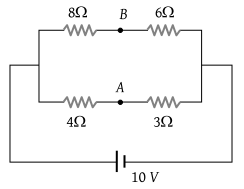# NEET Questions Solved

PMT - 2004

The potential difference between point A & B is(1) $\frac{20}{7}\text{\hspace{0.17em}\hspace{0.17em}}V$

(2) $\frac{40}{7}\text{\hspace{0.17em}\hspace{0.17em}}V$

(3) $\frac{10}{7}\text{\hspace{0.17em}\hspace{0.17em}}V$

(4) 0

(4) The given circuit is a balanced wheatstone bridge circuit. Hence potential difference between A and B is zero.

Difficulty Level:

• 21%
• 21%
• 17%
• 43%